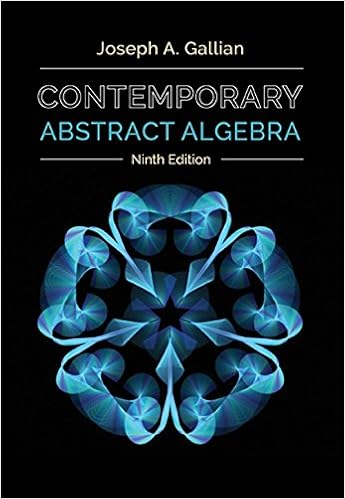# Analysis Sample Midterm - Analysis I section 5 SAMPLE...

• Test Prep
• 7
• 100% (1) 1 out of 1 people found this document helpful

This preview shows page 1 - 7 out of 7 pages.

##### We have textbook solutions for you!
The document you are viewing contains questions related to this textbook.The document you are viewing contains questions related to this textbook.
Chapter 16 / Exercise 16
Contemporary Abstract Algebra
GallianExpert Verified
Analysis I, section 5 - SAMPLE Midterm (10/22/2013) [100 marks]Name:Please justify all your answers, unless otherwise instructed. No calculators allowed.Duration: 75 minutes.1. Provide precise definition for the following objects (4 marks each):Completeness axiom of the real numbersCauchy sequence
##### We have textbook solutions for you!
The document you are viewing contains questions related to this textbook.The document you are viewing contains questions related to this textbook.
Chapter 16 / Exercise 16
Contemporary Abstract Algebra
GallianExpert Verified
2. Formulate the following theorems precisely (you do not have to prove them! 6 marks each)Squeeze theorem for real sequences (when the limit is a real number)Bolzano-Weierstrass theoremSequential Characterization of limits of real functionsIntermediate Value Theorem
3. Prove the Extreme Value theorem. (15 marks)
4. Decide which of the following statements are true and which are false. Prove the true ones and give counterex-amples to the false ones (6 marks each).
•••